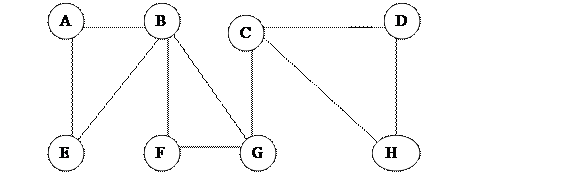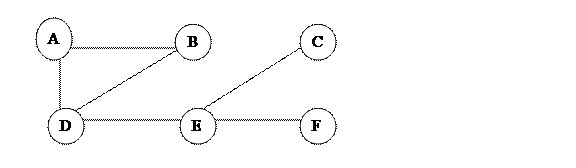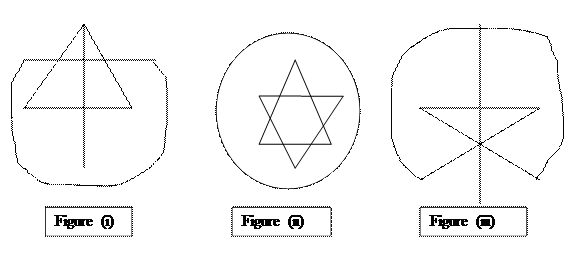Now You can search anything in sharecodepoint using your voice. Click on mic button.##### Sharecodepoint popular queries
Search results for Btech
Showing posts with label Btech. Show all posts

## B.Tech Information Technology Assignment 1- Discrete mathematics - Question Paper | lpu

Question 1. Consider the graph in fig one and obtain (a) the degree of every vertex and verify the handshaking lemma (b) all simple...

Question 1. Consider the graph in fig one and obtain (a) the degree of every vertex and verify the handshaking lemma
(b) all simple paths from A to G
(c) all trails (Distinct edges) from B to C
(d) d(A,C), The distance from A to C
(e)Diameter (G)Question 2. Consider the graph as shown in the fig one and obtain all the cycles, all cut points and bridges if any.

Question 3. Consider the subsequent graph. obtain the sub graph H(V’,E’) generated by:
(a) V’={B,C,D,E,F};(B) V’={A.C,E,G,H};(C) V’={B,D,E,H};(D) V’={C,F,G,H}
(b) Which of them are isomorphic and which are homomorphic?Question 4. Can a simple graph exists with 15 vertices every of degree five?

Question 5. Consider the multigraph G as shown in the figure (a)Which of them are connected? if they are not obtain the number of connected components .(b)which are cycle free .if they are not obtain the number of cycles (c)Which are loop free and Which are simple graphs?Question 6. Show that graph g with n vertices must have n-1 edges ?

Question 7. Draw 2 3-regular graphs with eight vertices ?

Question 8. consider every graph in G .Find the euler path or euler circuit if exists?and if does not why?
(see image in attachment )

Question 9. Consider every graph in G. obtain the Hamiltonian Path or circuit if exists and if does not why???

Question 10. Consider the complete graph Kn
(a) Find the no of m edges in Kn
(b) Find the degree of every vertex in Kn
(c) Find those values of n for which Kn is regular

Question 11. Suppose that G and G* ARE HOMOMORPHIC. Show that G is Eulerian if and only if G* is Eulerian.

## B.Tech Information Technology Assignment 1 - C++ - Question Paper | Lovely Professional University

ASSIGNMENT – 1 Classes And Objects Question 1. Distinguish ranging from the following: a) Classes and Objects b) Abstraction a...
ASSIGNMENT – 1 Classes And Objects

Question 1. Distinguish ranging from the following:
a) Classes and Objects
b) Abstraction and Encapsulations

Question 2. Discuss
a) Message Passing
b) Dynamic Binding
d) Object based languages
e) Object oriented languages

Question 3. What is meant by interface of a class and implementation of a class?

Question 4. Differentiate ranging from classes and structures with improper examples?

Question 5. Obtain Error:
```a) #include
#include
class a
{
private:
int x=20;
public:
void set(int a)
{
x=a;
}
int get()
{
return x;
}
void show()
{
cout<<”\nx= “ << get()<}};
```
```b)#include
#include
class a
{
private:
int x;
public:
void set(int a)
{
x=a;
}
int get()
{
return x;
}
void show()
{
cout<<”\nx= “ << get()<}
};
```
```c) #include
#include
class a
{
private:
int x;
public:
void show()
{
cout<<”\nx= “ <}
};
void main()
{
a obj;
obj.x=10;
obj.show();
}```
Question 6. Create a class to represent a bank account.It should include the following:

Data Members:
Name of account holder
Account number
kind of account
Balance account
Member Functions:
To initialize the data members with improper data
To deposit an account
To withdraw an amount afer checking the balance
To display details of account holder

Question 7. Write the output of subsequent programs:
```a)# include
#include
# include
void main()
{
char a;
clrscr();
a=getch();
cout<<”value of a=”<a=getche();
cout<<”value of a=”<a=getchar();
cout<<”value of a=”< }
```
```b)# include
# include
int a=7;
void main()
{
int i,a=10;
clrscr();
{
int a=20;
cout<<”In inner block value of a=”< cout<<” In inner block value of ::a=”<<::a;
}
cout<<”In outerr block value of a=”< cout<<” In outer block value of ::a=”<<::a;
for( i=0;i<=5;i++)
cout<<”value of i++=”<
for( i=0;i<=5;++i)
cout<<”value of ++i=”<getch();
}```
Question 8. Provide the classification of different access specifiers. How are they various from every other?

Question 9. There are various advantages of inline function, discuss them with suitable example
And if member functions are described within class declaration then those functions
are inline or not?

Question 10. Write down difference ranging from call by value,call by reference and call by address

Question 11.  Write down difference ranging from default arguments and general arguments with
Suitable example

## CSE415 : Compiler Design End Question Paper Question Paper | CSE 415 | Lpu Question Paper

A compiler is computer software that transforms computer code written in one programming language into another computer language. ...

A compiler is computer software that transforms computer code written in one programming language into another computer language.

1. what do you understand by longest pattern matching rule in LEX tool?
2. define a token
3. what do you understand by backtracking of a compiler. discuss in context with left factoring
4. what is left factoring and how left factoring is eliminated from the grammar?
5. what are the different conflicts that occur during shift-reduce parsing
6. give example of l-atributed definition
7. describe dependency graph in SDT
8. what are the various methods of implementing three address statements ?
9. what are the triplets prrferred over quadraples as intermediate code?
10. what are activation records?

PART-B

2 a) write short notes on i)buffer pairs ii)sentinentals
or
b) i)give an example of lexical error. how lexical errors are handled
ii)briefly explain about compiler construction tools

3 a)i)consider the g
a)what do you men by an ambigious grammar?consider the following grammar.
E-->E+E|E*E|1.
Check whether the grammar is ambigious for the input string:1+1*1.
Give an appropriate to rewrite the grammar to remove ambiguity.
OR
b)(i)Consider the context free grammar.
S-->SS+|SS*|a
and the string aa+a*.
a)Give a leftmost derivation for the string.
b)give the rightmost deivation for the string.
c)give the parse tree for the string.
d)is the grammar ambigious or unambigious ?justify.
(ii)Define a context free grammar with the help of an example.

Q4. a)consider the grammar with the folllowing translation rules and E as the start symbol.
E-->E*T (TE value =E value *T value).
IF (Evalue =t value).
T-->T&F (T value=T value+F.value).
IF (T value=F value).
F-->num(F.value=num.value).
Compute E.value for the root of the parse tree for expression:2#3&5#6&4.
b)(i)For the given syntax directed definitions draw the annotated parse trees for the expression
1*2*3*(4+5)n
PRODUCTIONS SEMANTIC RULES.
L->En L val=E.val
E->E1+T E.val=E1.val++T.val
E->T E.val=T.val
T->T1*F T.val=T1.val*F.val.
T->F T.val=F.val>
F->(E) F.val=E.val
F->digit F.val=digit*lexval.
(ii) for the given syntax directed definition draw the annotated parse trees for the expression : float
w,x,y
PRODUCTIONS SEMANTIC RULES
D->TL L.inh=T.type
T->int T.type=integer
T->float t.type=float

5 a)write three adress code,quadraple , triples, syntax tree and DAG for the the following
expressions:(consider operator procedure as per ANSIC)
i. a=b*-c+b*-c ii. -(a+b)*(c+d)+(a+b+c)
or
b)(i) write the three adress code for the following statement:
int a,b,d,i;
d=0;
for(i=0;i<10;i++)d+=a[i]*b[i];
ii)write the three adress code for the following code:
int fact(int n)
{
if n==0) return1;
else return (n*fact(n-1));
}

6 a)consider quicksort example pseudocoe,show downward-growing stack of activation record and
activation tree for quicksort example
quicksort(A,p,r){
if(p<r){
q<-partition(A,p,r)
quick sort(A,p,r)
quick sort (A,q+1,r)
}
}
partition(A,p,r){
a<-A[p]
i<-p-1
j<- r+1
while(true){
repeat
j<- j-1
until (a[j]<=x)
repeat
i<-i+1
until (A[i]>=x)
if(i<j)
swap(A[i],A[j])
else
return(j)
}
}
or
b i)consider following code:
int fib(n){
if(n==0) return 1;
if (n==1) return 1;
return fib(n-1)+fib(n-2);
}
draw the activation tree for n=5
ii) write short notes on following
i)activation trees ii)activation record

## ECE312 : Microprocessor Based System Design End Term Exam Question Paper | ECE 312 | LPU

Microprocessors—single-chip computers—are the building blocks of the information world. Their performance has grown 1,000-fold over th...

Microprocessors—single-chip computers—are the building blocks of the information world. Their performance has grown 1,000-fold over the past 20 years, driven by transistor speed and energy scaling, as well as by microarchitecture advances that exploited the transistor density gains from Moore's Law.

## ECE313 : Microprocessor End Term Exam Question Paper | ECE 313 | LPU

Microprocessor is defined as a silicon chip embedded with a Central Processing Unit or CPU. It is also referred to as a computer's...

Microprocessor is defined as a silicon chip embedded with a Central Processing Unit or CPU. It is also referred to as a computer's logic chip, micro chip, and processor. Advantages of Microprocessors is that these are general purpose electronic processing devices which can be programmed to execute a number of tasks.

## ECE312 : Microprocessor Based System Design End Term Exam Question Paper | ECE 312 | LPU

A microprocessor is an electronic component that is used by a computer to do its work. It is a central processing unit on a single int...

A microprocessor is an electronic component that is used by a computer to do its work. It is a central processing unit on a single integrated circuit chip containing millions of very small components including transistors, resistors, and diodes that work together.

## CSE320 : Software Engineering Mid Term Exam Question Paper | CSE 320 | LPU

Software engineering is a field of engineering, for designing and writing programs for computers or other electronic devices.

## CSE320 : Software Engineering End Term Exam Question Paper | CSE 320 | LPU

Field Overview. Computer software engineers apply the principles and techniques of computer science, engineering, and mathematical analysi...
Field Overview. Computer software engineers apply the principles and techniques of computer science, engineering, and mathematical analysis to the design, development, testing, and evaluation of the software and the systems that enable computers to perform their many applications.